Model Test Paper

# Model Test Paper

Test Description

## 35 Questions MCQ Test Mathematics Olympiad Class 4 | Model Test Paper

Model Test Paper for Class 4 2022 is part of Mathematics Olympiad Class 4 preparation. The Model Test Paper questions and answers have been prepared according to the Class 4 exam syllabus.The Model Test Paper MCQs are made for Class 4 2022 Exam. Find important definitions, questions, notes, meanings, examples, exercises, MCQs and online tests for Model Test Paper below.
Solutions of Model Test Paper questions in English are available as part of our Mathematics Olympiad Class 4 for Class 4 & Model Test Paper solutions in Hindi for Mathematics Olympiad Class 4 course. Download more important topics, notes, lectures and mock test series for Class 4 Exam by signing up for free. Attempt Model Test Paper | 35 questions in 70 minutes | Mock test for Class 4 preparation | Free important questions MCQ to study Mathematics Olympiad Class 4 for Class 4 Exam | Download free PDF with solutions
 1 Crore+ students have signed up on EduRev. Have you?
Model Test Paper - Question 1

### Which of the given rectangle has the largest perimeter?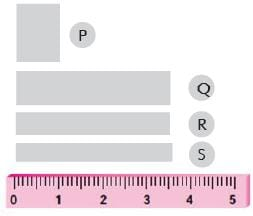Model Test Paper - Question 2

### Which one of the following has NO line of symmetry ?

Model Test Paper - Question 3

### Find the values of P and Q respectively.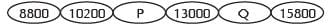Detailed Solution for Model Test Paper - Question 3

The common difference between each consecutive term is 1400.

So P =10200+1400 = 11600

Q = 13000+1400 = 14400

Model Test Paper - Question 4

Which house has the largest value ?

Model Test Paper - Question 5

12 friends met at a party. Each friend shook hands with each other exactly once. Number of handshakes took place is _____.

Detailed Solution for Model Test Paper - Question 5

The first person shook hands with 11 remaining people, the second person also shook hands with 11 people, but we count 10, as the hand shake with the first person has already been counted.

Then add 9 for the third person, 8 for the fourth one & proceeding in this fashion

We get: 11 + 10 + 9 + 8 + 7 + 6 + 5 + 4 + 3 + 2 + 1 = 66.

Model Test Paper - Question 6

Riya is 17th from the front in a queue. She is 19th from the end. Then how many students are there in the queue?

Model Test Paper - Question 7

Removing which of the following square will result no change in the perimeter of the given figure?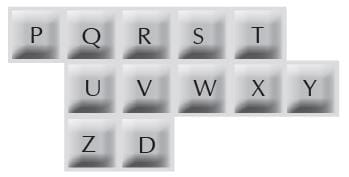Model Test Paper - Question 8

If there are 6 cells around one cell, 8 cells around two cells in a row, then number of cells around a row of three cells is _____.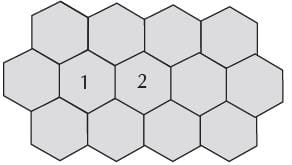Model Test Paper - Question 9

Rohan wants to move just one matchstick to make the equation correct. Which part will he take out ?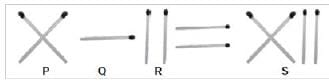Model Test Paper - Question 10

Find the missing number in Fig. (X).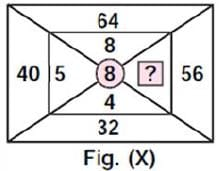Model Test Paper - Question 11

Which of the following is equivalent to “five lakhs one hundred and nine”?

Model Test Paper - Question 12

The given bar graph shows the height of five children. What is the difference between the height of the tallest child and the shortest child ?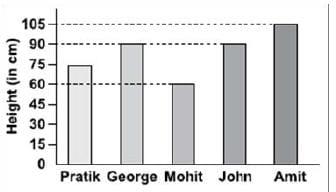Model Test Paper - Question 13

The perimeter of the star equals the perimeter of the hexagon (all sides are equal). If each side of the hexagon is 20 cm long, then each side of the star is ____.​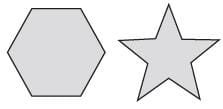Detailed Solution for Model Test Paper - Question 13

Perimeter is the sum of all boundaries of a figure.

Hexagon has 6 sides so permieter will be 6 x side length = 6x20 = 120 cm

Now since star and hexagon have same perimeter, Star has 10 sides

so perimeter will be

10 x side length = 120

side length = 120/10 = 12cm

Model Test Paper - Question 14

If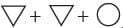= 120 and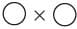= 100, then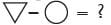Detailed Solution for Model Test Paper - Question 14

Circle * Circle = 100

Let Circle = y

y * y = 100

y2 = 100

y = 10

Triangle + Triangle + Circle = 120

Let Triangle = x

x + x + 10 = 120

2x = 110

x = 55

Therefore, Triangle - Circle

= 55 - 10 = 45

Model Test Paper - Question 15

Which of the following shows all the factors of 30?

Model Test Paper - Question 16

Find the value of Y to make the expression true. 8000 = 50 × 80 × Y

Model Test Paper - Question 17

Arrange the boxes in order, beginning from the heaviest to lightest.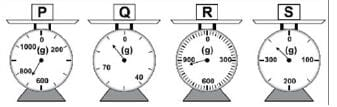Model Test Paper - Question 18

How much does the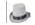cost ?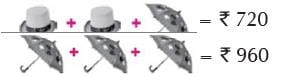Model Test Paper - Question 19

Which fraction of the given figure is unshaded?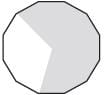Model Test Paper - Question 20

Where will the minute hand point after 1 hour 45 minutes from the time shown on the clock ?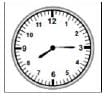Model Test Paper - Question 21

Roman numeral equivalent to 89 is ______.

Model Test Paper - Question 22

What is the weight of each box?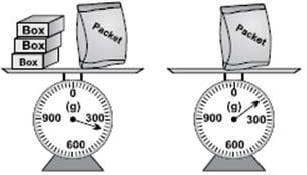Model Test Paper - Question 23

In which of the following dotted lines shows a line of symmetry?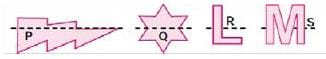Detailed Solution for Model Test Paper - Question 23

The line in Q only, divides the figure in two equal halves

Model Test Paper - Question 24

The product X = 2 × 2 × 3 × 5 × 7 × 7 × 11 × 13 × 17 × 19 is not divisible by a number. Which is the number?

Model Test Paper - Question 25

Find the value of X.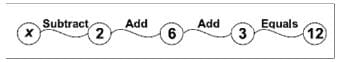Detailed Solution for Model Test Paper - Question 25

X - 2 + 6 + 3  = 12

X = 5

Model Test Paper - Question 26

Jenny and Henry sold together 90 chocolates during a sales promotion. Henry sold twice as many chocolates than Jenny. How many chocolates did Jenny sell?

Model Test Paper - Question 27

The capacity of a small container is 380 ml and the capacity of a big container is 1250 ml. If Aakash uses 8 small containers and 1 big container of water to fill up an empty tank, then what is the capacity of the tank?

Model Test Paper - Question 28

Priya is practicing for a race. She uses the given map to find the possible routes. The route must start from her home and end at the park. She wants to run exactly 7 km. Which of the following is the best route?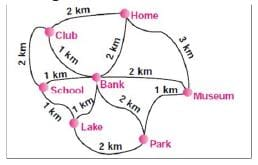Model Test Paper - Question 29

The length of a square field is 480 m. Vishal runs 5 rounds around the field. Find the total distance ran by Vishal.

Model Test Paper - Question 30

Looking at the adjoining figure, how many ancestors did Garima had four generations ago?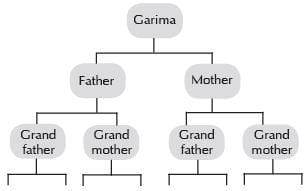Model Test Paper - Question 31

Arshan packed 726 burgers into packets of 3. He sold them at Rs. 2 per packet. How much did he get in all?

Model Test Paper - Question 32

There are 200 people in a hall. They are divided equally into 7 groups of adults and 3 groups of children. If there are 2 adults in each group, then how many children are there in each group ?

Detailed Solution for Model Test Paper - Question 32

The correct answer is d

Let adult number be A and children number be C

7A + 3C = 200……Eq..1

7 × 2 + 3C = 200.. Eq..2

14 + 3C = 200

3C = 200 - 14

3C = 186

C = 186/3

C = 62

There are 62 children in each of the 3 groups

Model Test Paper - Question 33

Three friends, Payal, Latika and Megha are at the corners of a rectangular field. At which point should they meet so that the distances they walk to that point is minimized?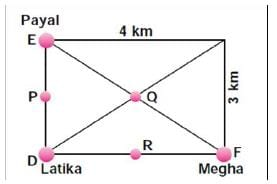Model Test Paper - Question 34

Prerna bought 80 peaches. She found out that out of every 10 peaches, 2 are rotten and had to be thrown away. How many peaches in the end are left with her?

Model Test Paper - Question 35

In a grazing field there are 60 goats, 30 deers and 10 children. How many legs are there in the field?

Detailed Solution for Model Test Paper - Question 35

Goats and deers have 4 legs each. So number of legs of all goats and deers is

60+30 =90 x 4 = 360

and children (human) have 2 legs each, so

10 x 2 = 20 legs

total number of legs = 360+20 = 380

## Mathematics Olympiad Class 4

4 videos|39 tests
 Use Code STAYHOME200 and get INR 200 additional OFF Use Coupon Code
Information about Model Test Paper Page
In this test you can find the Exam questions for Model Test Paper solved & explained in the simplest way possible. Besides giving Questions and answers for Model Test Paper, EduRev gives you an ample number of Online tests for practice

## Mathematics Olympiad Class 4

4 videos|39 tests